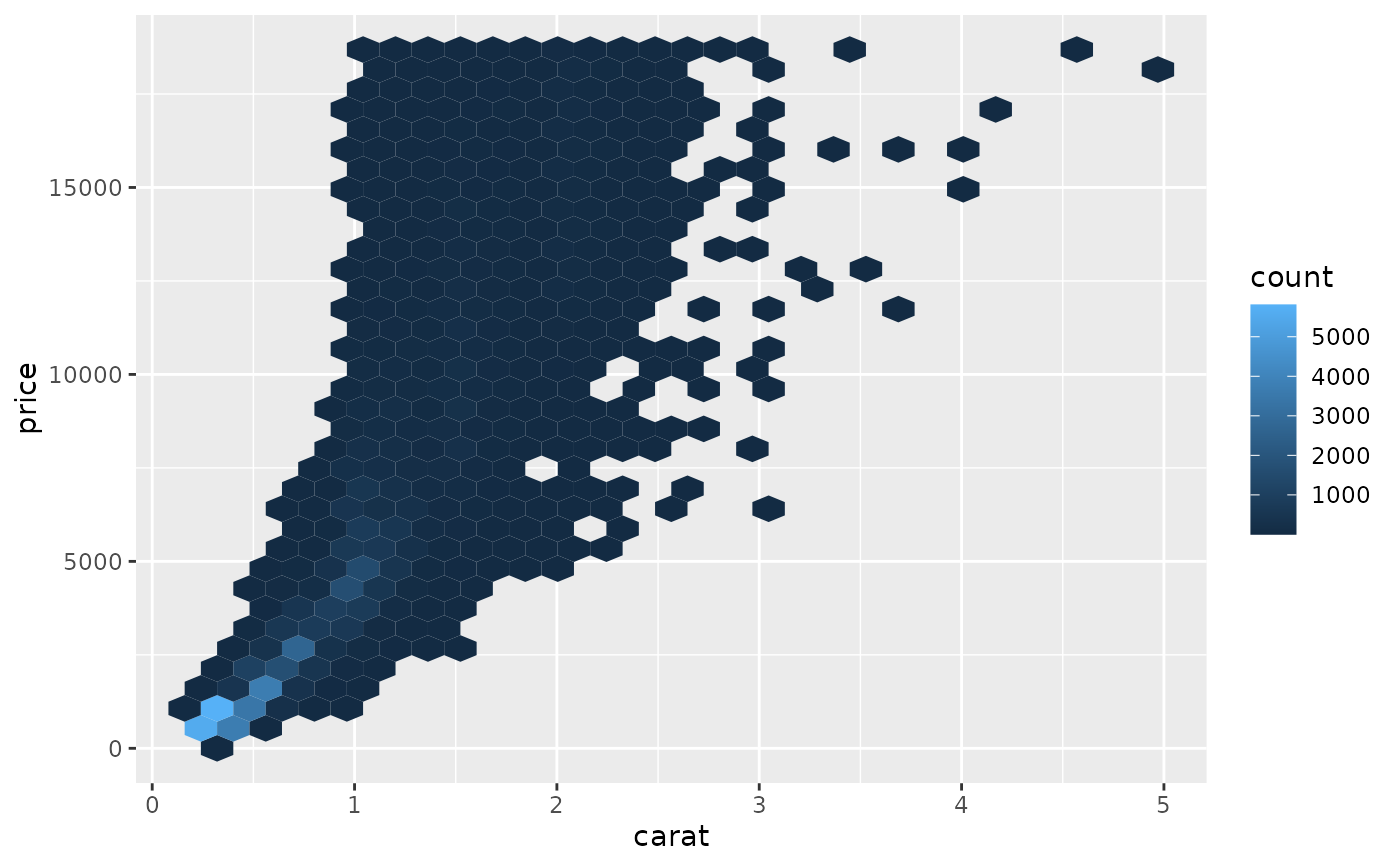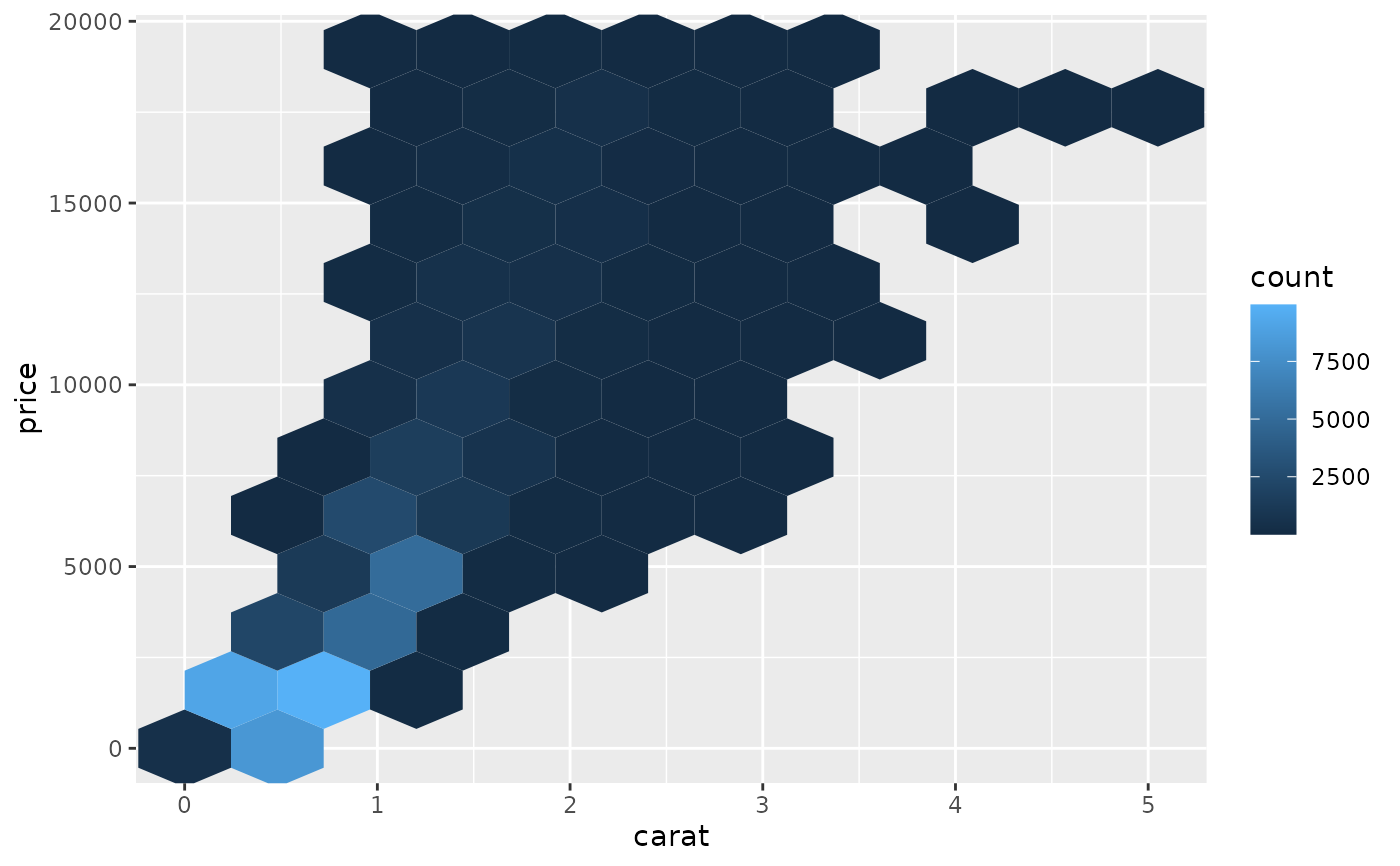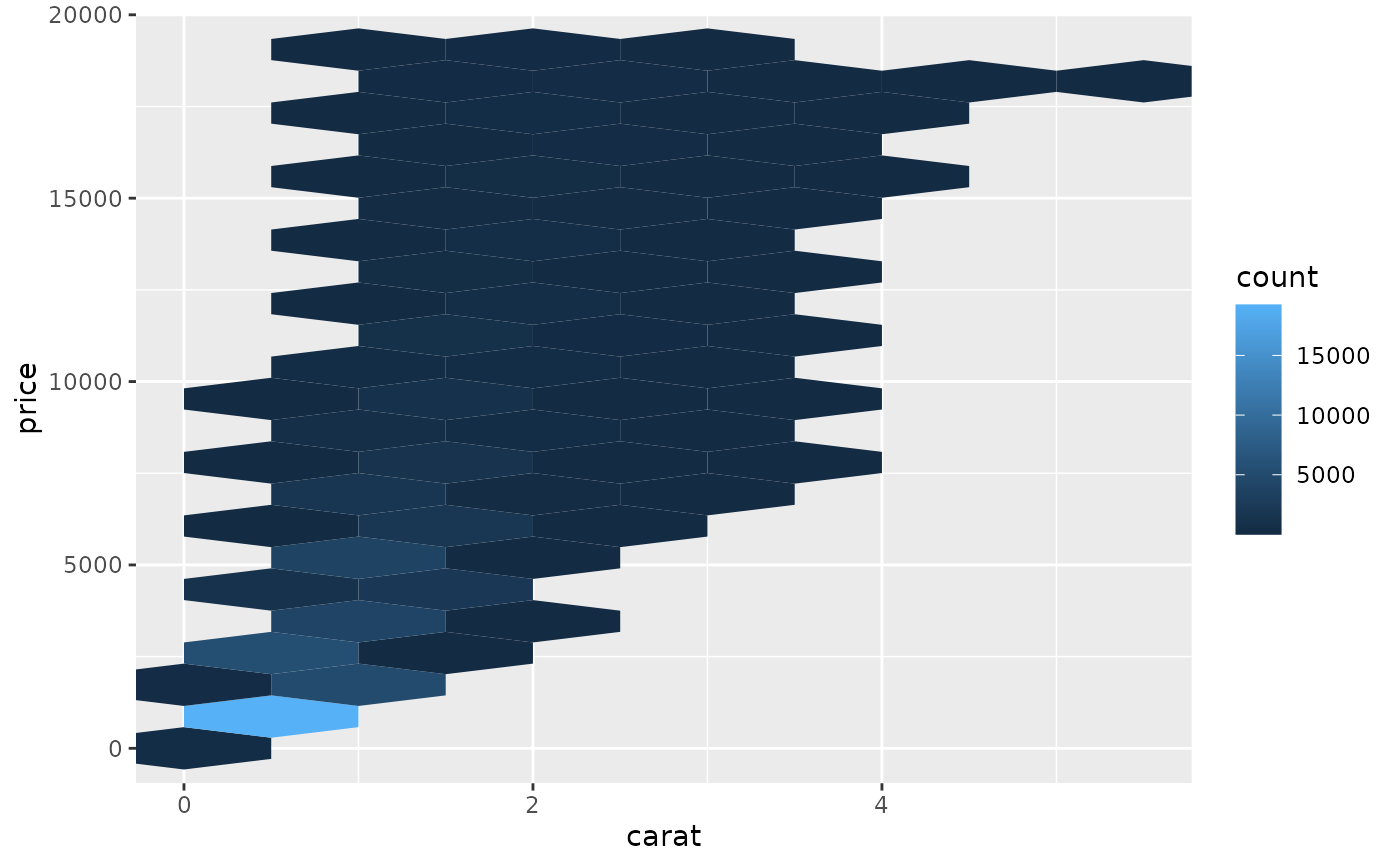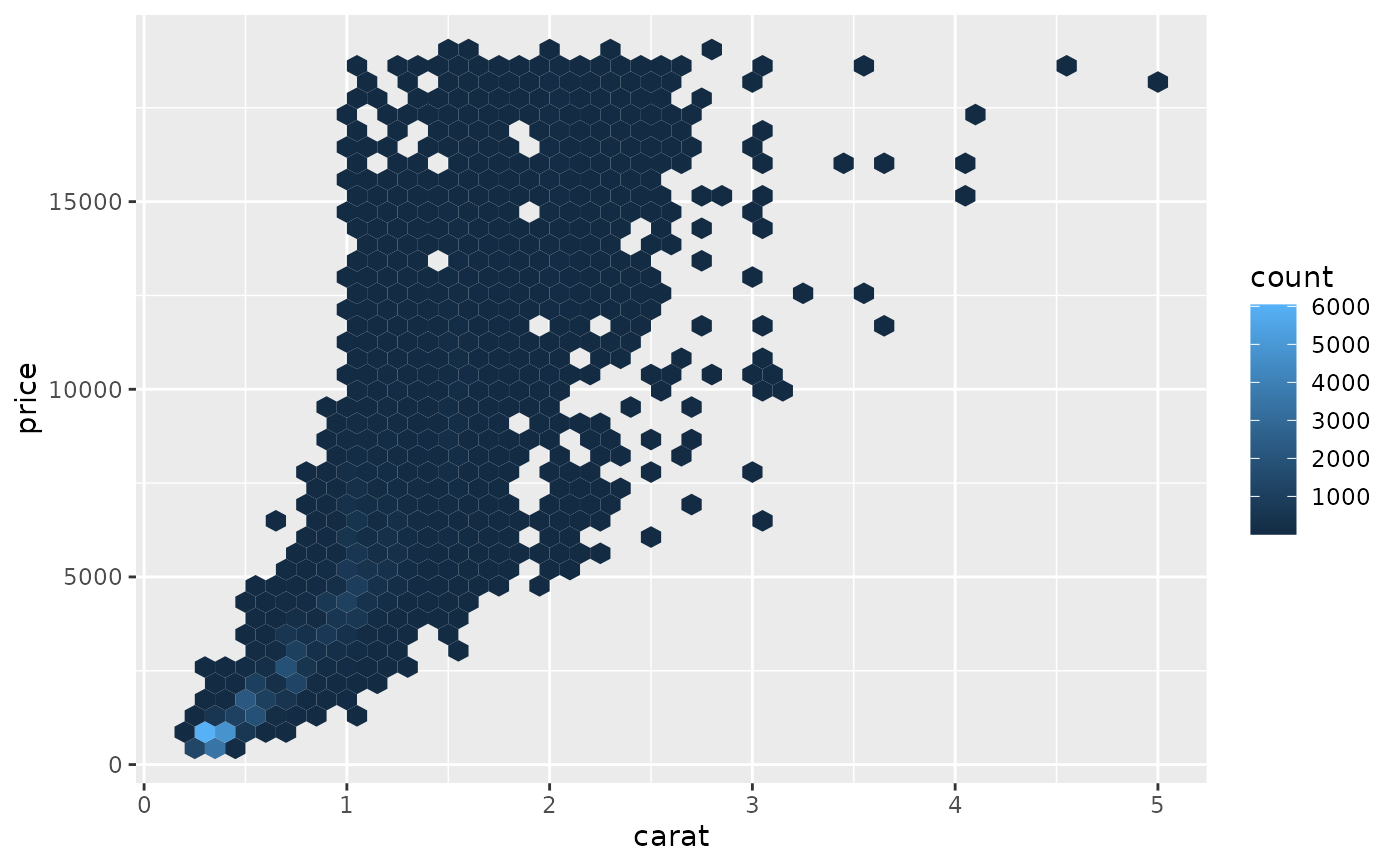Divides the plane into regular hexagons, counts the number of cases in each hexagon, and then (by default) maps the number of cases to the hexagon fill. Hexagon bins avoid the visual artefacts sometimes generated by the very regular alignment of geom_bin2d().

## Usage

geom_hex(
mapping = NULL,
data = NULL,
stat = "binhex",
position = "identity",
...,
na.rm = FALSE,
show.legend = NA,
inherit.aes = TRUE
)

stat_bin_hex(
mapping = NULL,
data = NULL,
geom = "hex",
position = "identity",
...,
bins = 30,
binwidth = NULL,
na.rm = FALSE,
show.legend = NA,
inherit.aes = TRUE
)

## Arguments

mapping

Set of aesthetic mappings created by aes(). If specified and inherit.aes = TRUE (the default), it is combined with the default mapping at the top level of the plot. You must supply mapping if there is no plot mapping.

data

The data to be displayed in this layer. There are three options:

If NULL, the default, the data is inherited from the plot data as specified in the call to ggplot().

A data.frame, or other object, will override the plot data. All objects will be fortified to produce a data frame. See fortify() for which variables will be created.

A function will be called with a single argument, the plot data. The return value must be a data.frame, and will be used as the layer data. A function can be created from a formula (e.g. ~ head(.x, 10)).

position

Position adjustment, either as a string naming the adjustment (e.g. "jitter" to use position_jitter), or the result of a call to a position adjustment function. Use the latter if you need to change the settings of the adjustment.

...

Other arguments passed on to layer(). These are often aesthetics, used to set an aesthetic to a fixed value, like colour = "red" or size = 3. They may also be parameters to the paired geom/stat.

na.rm

If FALSE, the default, missing values are removed with a warning. If TRUE, missing values are silently removed.

show.legend

logical. Should this layer be included in the legends? NA, the default, includes if any aesthetics are mapped. FALSE never includes, and TRUE always includes. It can also be a named logical vector to finely select the aesthetics to display.

inherit.aes

If FALSE, overrides the default aesthetics, rather than combining with them. This is most useful for helper functions that define both data and aesthetics and shouldn't inherit behaviour from the default plot specification, e.g. borders().

geom, stat

Override the default connection between geom_hex() and stat_binhex().

bins

numeric vector giving number of bins in both vertical and horizontal directions. Set to 30 by default.

binwidth

Numeric vector giving bin width in both vertical and horizontal directions. Overrides bins if both set.

## Aesthetics

geom_hex() understands the following aesthetics (required aesthetics are in bold):

• x

• y

• alpha

• colour

• fill

• group

• linetype

• linewidth

Learn more about setting these aesthetics in vignette("ggplot2-specs").

## Computed variables

These are calculated by the 'stat' part of layers and can be accessed with delayed evaluation.

• after_stat(count)
number of points in bin.

• after_stat(density)
density of points in bin, scaled to integrate to 1.

• after_stat(ncount)
count, scaled to maximum of 1.

• after_stat(ndensity)
density, scaled to maximum of 1.

stat_bin2d() for rectangular binning

## Examples

d <- ggplot(diamonds, aes(carat, price))
d + geom_hex()# \donttest{
# You can control the size of the bins by specifying the number of
# bins in each direction:
d + geom_hex(bins = 10)d + geom_hex(bins = 30)# Or by specifying the width of the bins
d + geom_hex(binwidth = c(1, 1000))d + geom_hex(binwidth = c(.1, 500))# }